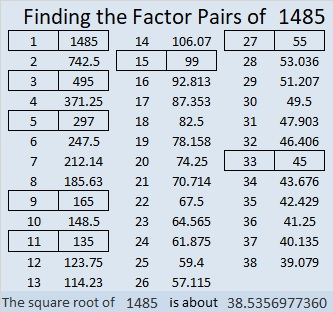# 1485 Sticky Lollipop Mystery

Contents

### Today’s Puzzle:

Lollipops can be very sticky. Will the logic needed for this puzzle be a sticky mess, or will you be able to lick it? That’s the mystery. Good luck!### Factors of 1485:

• 1485 is a composite number.
• Prime factorization: 1485 = 3 × 3 × 3 × 5 × 11, which can be written 1485 = 3³ × 5 × 11
• 1485 has at least one exponent greater than 1 in its prime factorization so √1485 can be simplified. Taking the factor pair from the factor pair table below with the largest square number factor, we get √1485 = (√9)(√165) = 3√165
• The exponents in the prime factorization are 3,1 and 1. Adding one to each exponent and multiplying we get (3 + 1)(1 + 1) (1 + 1) = 4 × 2 × 2 = 16. Therefore 1485 has exactly 16 factors.
• The factors of 1485 are outlined with their factor pair partners in the graphic below.### More about the Number 1485:

Did you notice: (27)(55) = 1485? That means that
(54)(55)/2 = 1485 so 1485 is the 54th triangular number.

1485 is the hypotenuse of a Pythagorean triple:
891-1188-1485 which is (3-4-5) times 297.

Of the ten numbers from 1480 to 1489, four are prime numbers and have exactly 2 factors. Three of the ten numbers have exactly 16 factors, namely 1480, 1482, and 1485. No smaller set of three numbers with sixteen factors are as close together as these three are!

This site uses Akismet to reduce spam. Learn how your comment data is processed.